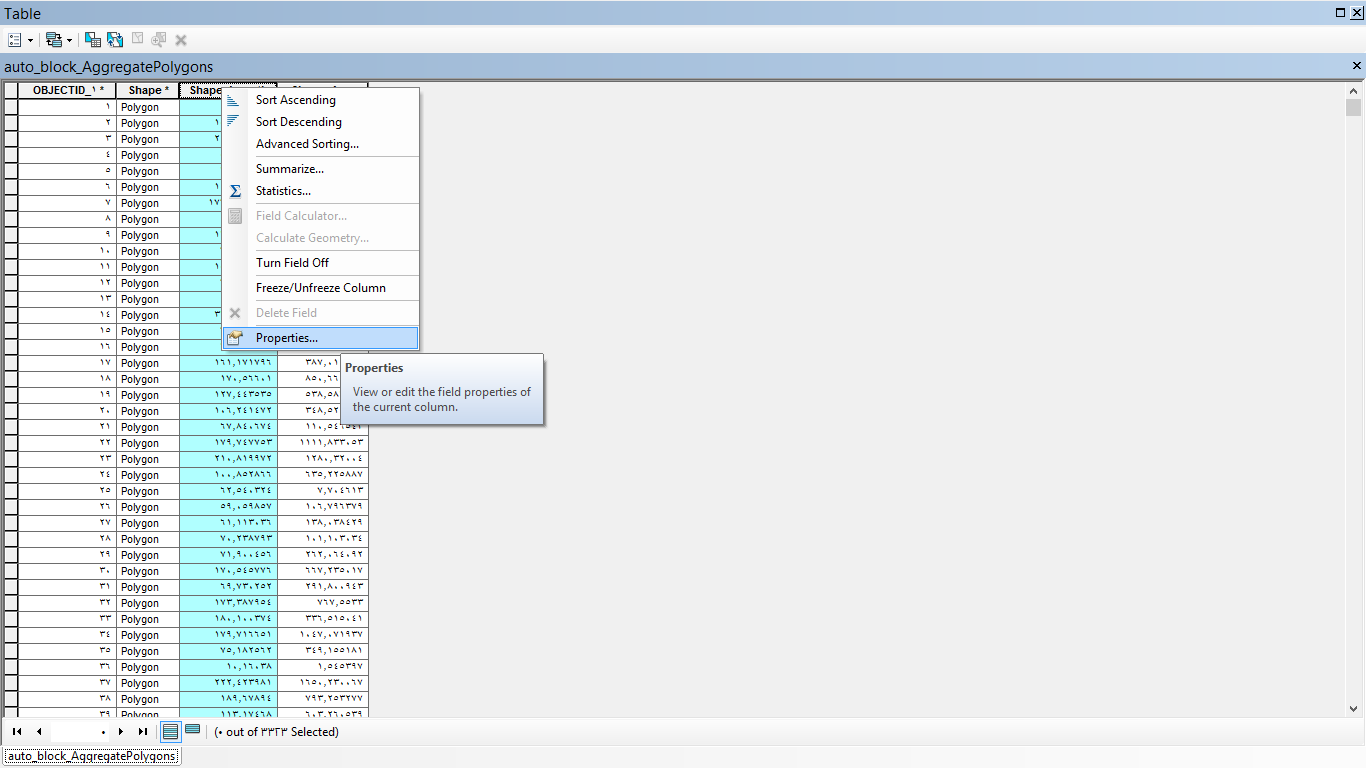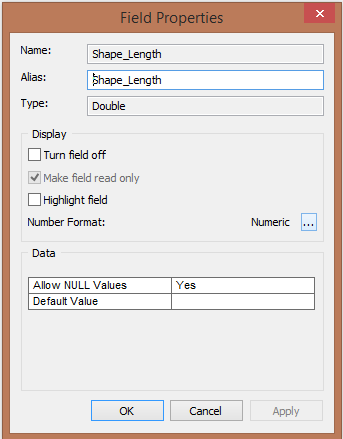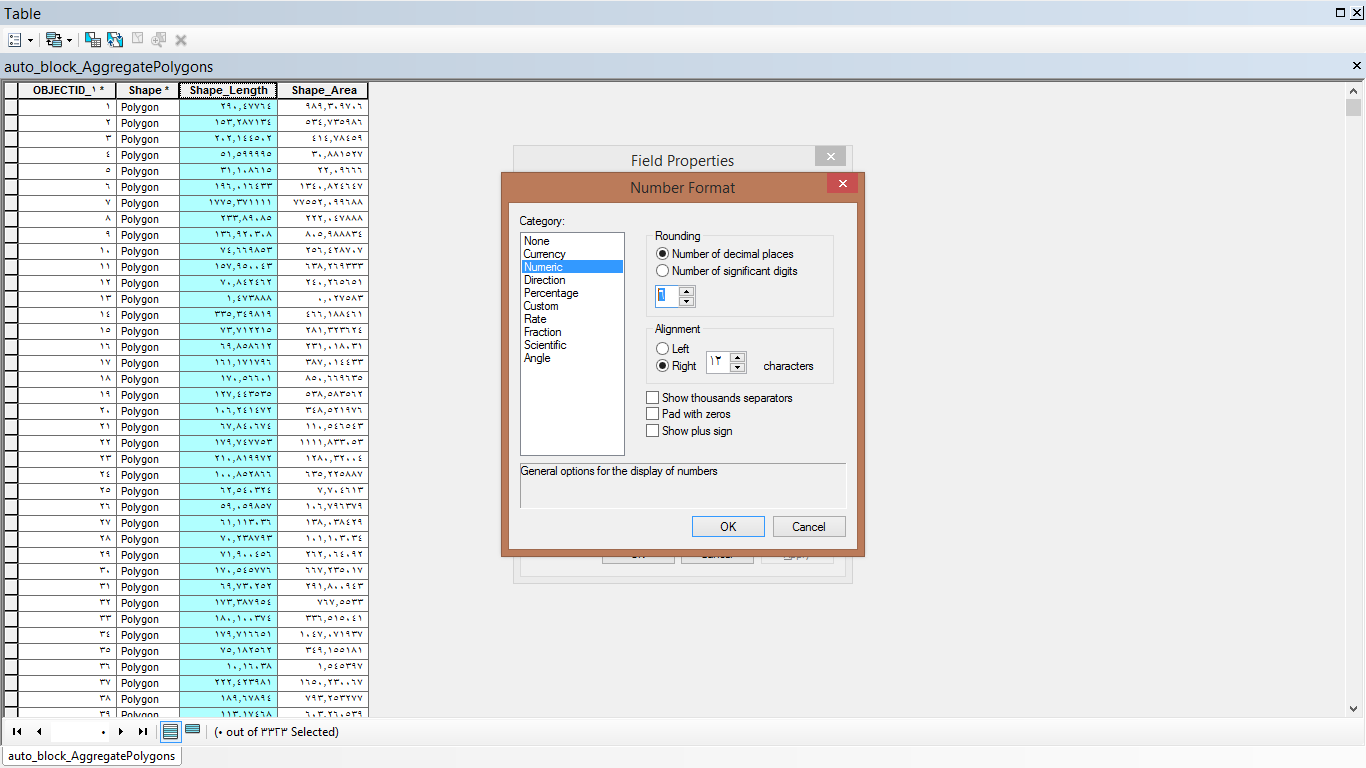# Problem with precision and scale in new field

2648
16
07-18-2016 12:28 PMNew Contributor

Hello GeoNet community,

I have a question regarding precision and scale of a new field. I am working on making a new field where I need total of 18 digits including 8 digits after decimal. For example the math is

900000000 + 1111*10000 + 1111*1 + 11111111/100000000

I need the result is 911111111.11111111

But, it shows 911111111.11111116

FYI, I have used Double and changed the precision and scale when I add the field. I will be appreciated if anyone has some ideas to explore.

Thanks

Reja

16 RepliesRegular Contributor II

In attribute table

right click on the top of the field that you want to set specific precision . and click on propertiesInside Field Properties window. click on dots icon  ( ... ) it is beside Numeric.choose from The left Numeric, then choose number of decimal places . and set it to be 8 as you want.New Contributor

Thanks Anter! I did this part too. However, the problem is still unsolved. I don't know why this is rounding up.Regular Contributor II

you said " I need the result is 911111111.11111111

But, it shows 911111111.11111116"

Both precision is 8

The difference in value not in precision.New Contributor

No, actually the scale is 8, precision is 17. Do you have any idea then why the value is changed as I used the scale is 8?byMVP Honored Contributor

I believe  the maximum number of digits stored internally in a double is 17 digits, so you may have exceeded the limits of what it is capable of displaying reliably.  At least this is true in .Net.

"Because some numbers cannot be represented exactly as fractional binary values, floating-point numbers can only approximate real numbers.

All floating-point numbers also have a limited number of significant digits, which also determines how accurately a floating-point value approximates a real number. A Double value has up to 15 decimal digits of precision, although a maximum of 17 digits is maintained internally. "Regular Contributor II

I calculate the same Yusuf math in my calculator

the result not exceed  8 decimal digits of precision.

the result is 911111111.11111111Regular Contributor II

Yusuf, How did you change the scale?byMVP Esteemed Contributor

You have reached the limits of floating point precision.  The only other build in math module that support higher representation is Python's Decimal class and I think the mpmath module... both of which can't be used in esri products.  You have an alternate solution if this is purely for display purposes... that is to concatenate string representations of the integer and decimal portions of the number.  Your number is being represented as best it can and won't affect calculations

91111111.1111111

>>> a = 911111111.11111111

>>> a

911111111.1111112

>>> a-a

0.0Regular Contributor II

Dan, your viewpoint might to be right, I also thought about that .

But

Md Yousuf Reja, wrote in the end of result number 6 not 2.

 mdyousufreja wrote:Hello GeoNet community,I have a question regarding precision and scale of a new field. I am working on making a new field where I need total of 18 digits including 8 digits after decimal. For example the math is900000000 + 1111*10000 + 1111*1 + 11111111/100000000I need the result is 911111111.11111111But, it shows 911111111.11111116